# About correlation coefficient and Correlation Coefficient Monitor

Hello!
In this article, we will introduce you to Hinemos Correlation Coefficient Monitor along with some explanation about the term “correlation coefficient”.  Some part may sound like some college mathematics lecture, but please bear with us.

## １．Definition of a correlation coefficient

So what is a correlation coefficient anyways?
Correlation means that “two or more things have a mutual relationship”.
When one thing changes in tandem with another’s change, you can say “there is a correlation between these two”.
A correlation coefficient is a measure of such a correlation.
It assumes a value in the range from -1 to +1. The closer to +1, the stronger the positive correlation is (when one thing increases, other the increases, too), and the closer to -1, the stronger the negative correlation is (when one thing increases, the other decreases).

Now, let’s check the definition of a correlation coefficient.
The general definition of a correlation coefficient is as follows.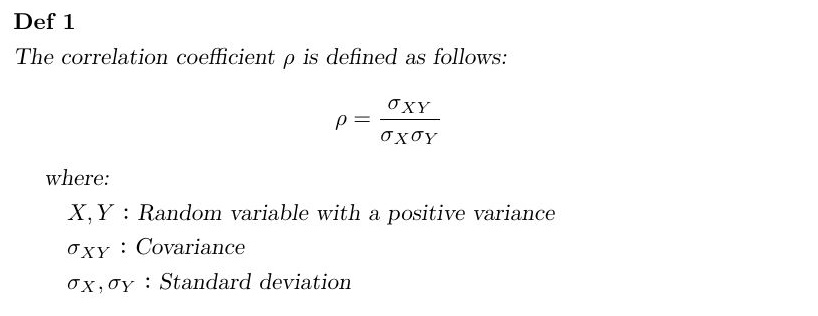I’m pretty sure that that didn’t ring any bells with any of you.
Some of you may recognize the terms  “covariance” and “standard deviation”, but terms like ”random variable” might not be familiar unless you have studied mathematical statistics. Why I am using such a complex term, I cannot explain since ❝there is not enough space❞ -Pierre de Fermat. If you are interested, please consider looking at Wikipedia or buying books on mathematical statistics written for university students. You can find more incomprehensible terms such as Lebesgue integral etc.

Ok, let’s change the definition above into a more comprehensible one by adding some conditions.
Specifically, by changing the number of data pieces to a finite number so that you can calculate using the summation method.
Shown below is the new definition.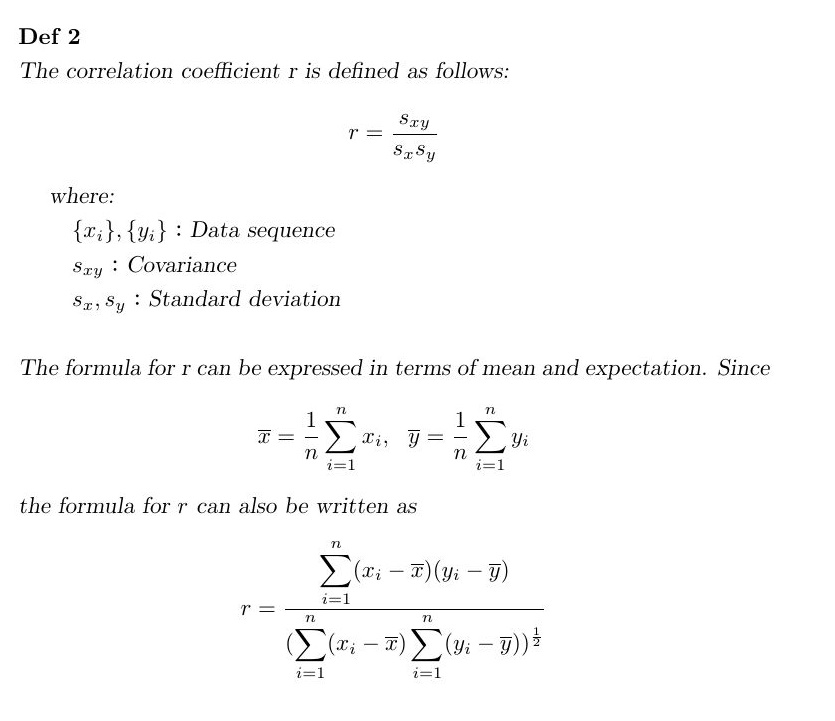The data column, used instead of a random variable here, is a collection of data used for calculating a correlation coefficient.
The second formula looks complicated, but we can probably manage to solve it somehow if we just have the necessary data.
This formula is more common since the finite number of data is more often used to calculate a correlation coefficient.
In fact, Hinemos Correlation Coefficient Monitor calculates a correlation coefficient using a finite number of collected data to judge whether the calculated value is within the threshold or not.

## ２．Correlation Coefficient Monitor

We have checked the definition of a correlation coefficient, but you may not be so sure how it is applied in the monitor setting of Hinemos.
You will need to use a correlation coefficient, which is basically a measure used for data analysis when you wish to clarify the relationship between two sets of data.
Hinemos Correlation Coefficient Monitor is used to monitor the relationship between two sets of collected data.
To give you a simple example, we configured the Correlation Coefficient Monitor setting shown below.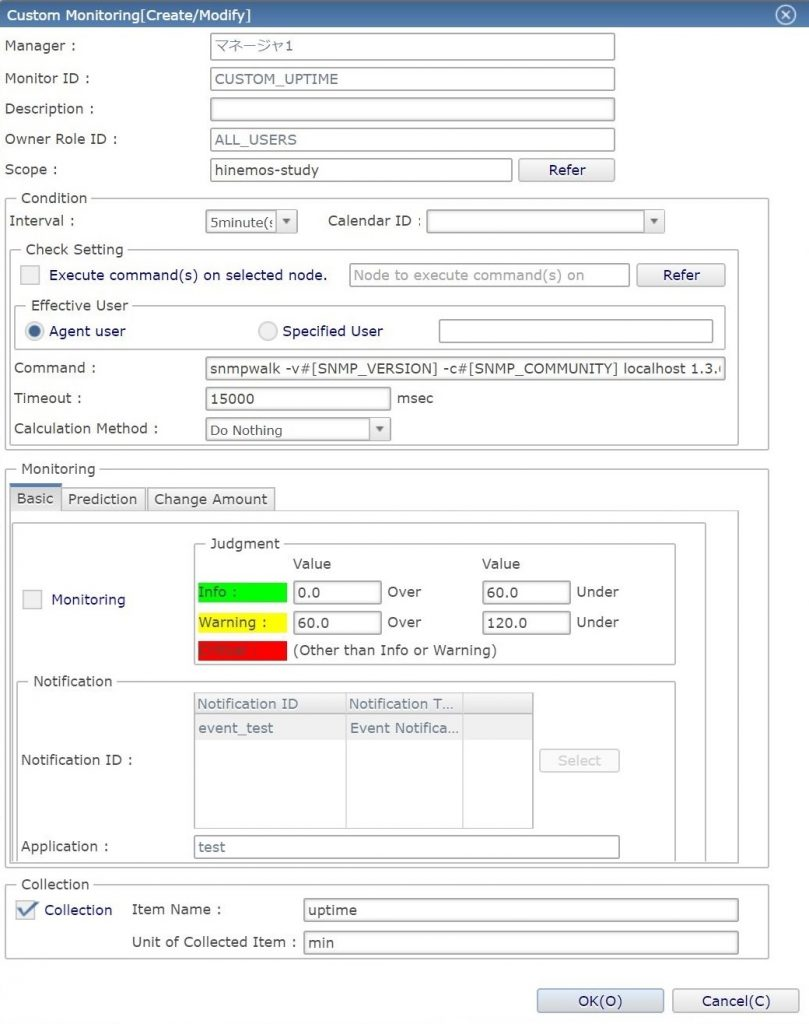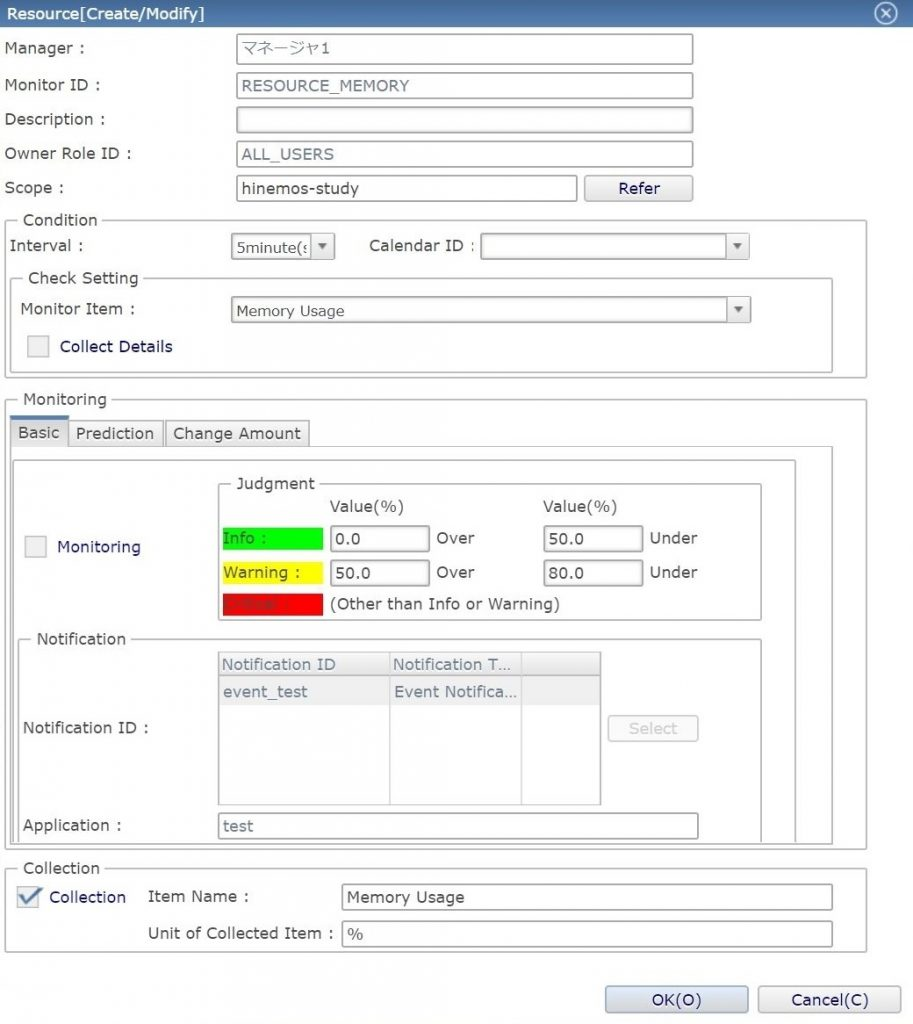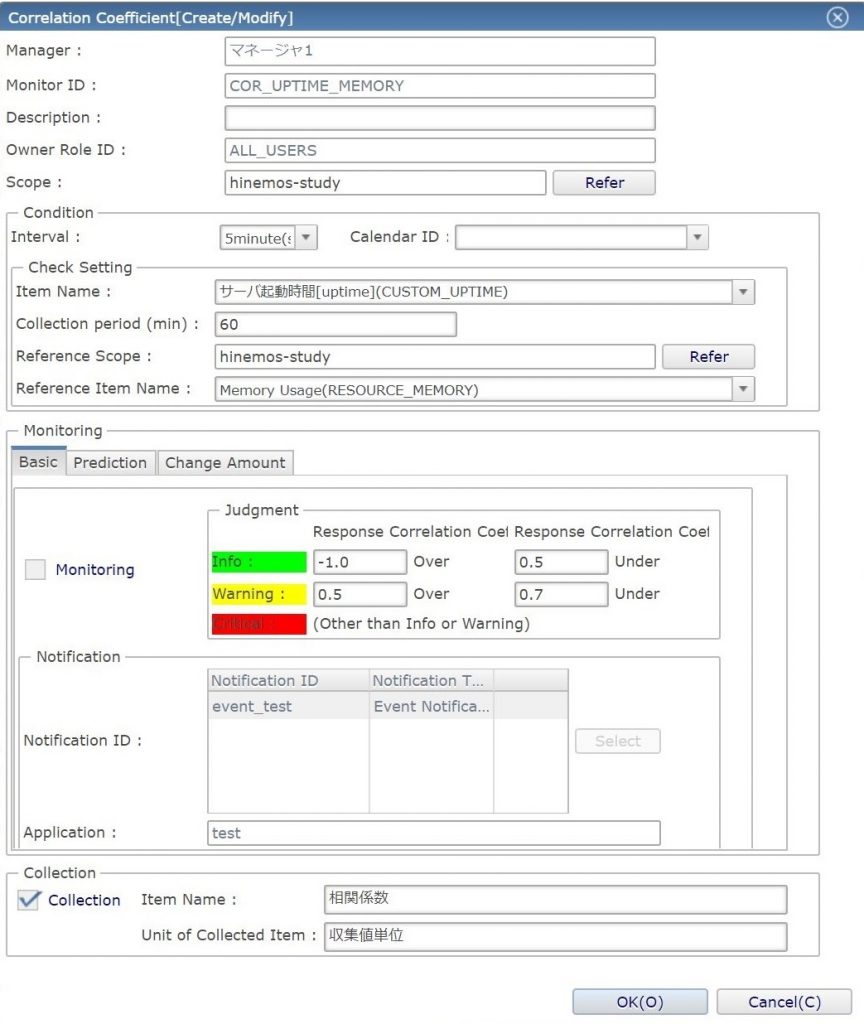While Custom Monitor is set to collect server startup time data (refer to this article for more details）, Correlation Coefficient Monitor is set to monitor the correlation coefficient between server startup time and memory utilization.
Since Correlation Coefficient Monitor cannot calculate a correlation coefficient without collected data, make sure to check the “Collect” checkbox in the two monitor settings for correlation coefficient calculation!

You can say that memory utilization will increase as time passes when there is a positive correlation between server startup time and memory utilization. In such a case, the priority level of monitor results will be judged as “Critical” since this may be due to a server problem.
Roughly speaking, when the absolute value of the correlation coefficient is 0.7 or above, the correlation can be judged as strong. Therefore, Hinemos is set to judge the priority of monitor results as “Critical” if the value is 0.7 or above.

Now, let’s check the monitor results.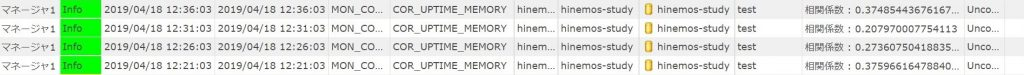The priority of the monitor results in the image above is judged as “Info”.
The correlation coefficients are less than 0.4. That means there would be no strong correlation between the time elapsed and memory utilization if we do nothing to it.
Next, let’s see what will happen if we increase memory utilization of the monitored server.

The results of the correlation coefficient monitoring performed while memory utilization is increasing are shown below.
You can see the correlation coefficient increased over time since memory utilization increased as time passed.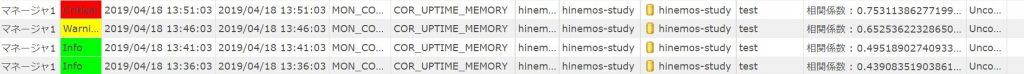The results of the correlation coefficient monitoring performed while memory utilization was kept high after it stopped increasing are shown below.
You can see the correlation coefficient decreased over time since memory utilization no longer increased even if time passed.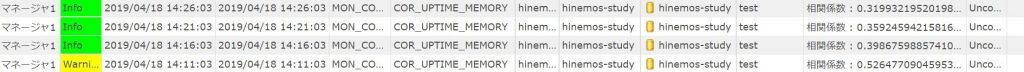As you can see, Correlation Coefficient Monitor is useful when you examine the relationship between two sets of collected data, not the collected data themselves.

## ３．Conclution

In the case above, we used a correlation coefficient between relatively easy-to-understand data items – server startup time and memory utilization – to judge the priority of monitor results.
If you just need to compare the time and the collected data, you can perform similar monitoring and judge the priority of its results using the change amount monitor.
The benefit of using Correlation Coefficient Monitor is that you can monitor the correlation between the collected data 1 and 2 during a particular period of time (specified data collection period).
In addition, there is almost no restriction on what data to collect, since you can use Custom Monitor to set data to collect.
If you keep these points in mind, you will be able to make more use of Correlation Coefficient Monitor.

That’s it for today.
And as always, thanks for reading.

## ご意見やお問い合わせについて

この記事へのご意見やお問い合わせについては、こちら（お問い合わせフォーム）もしくは
Hinemos営業担当【hsd-sales@atomitech.jp】までご連絡ください。

※お問い合わせフォームからご連絡の場合には、お問い合わせ内容で「Hinemosについて」を選択し、
本文の冒頭に「Hinemos研究日記_【タイトル名】」とご記載ください。
※メールアドレスへご連絡の場合には、件名を「Hinemos研究日記_【タイトル名】」でご連絡ください。